# RS Aggarwal Solutions for Class 10 Maths Chapter 3 Linear Equations in Two Variables Exercise 3F

RS Aggarwal Solutions for Class 10 Maths Chapter 3 Linear Equations in Two Variables, contains solutions for exercise 3F questions. This exercise helps students to get master on solving simultaneous linear equations using various methods and real-life problems. BYJU’s bring to you the R S Aggarwal Solutions for Class 10 Mathematics, providing detailed explanations to all the questions enlisted under exercises 3f. Students can download these RS Aggarwal Solutions of Class 10 for free and start practicing.

## Download PDF of RS Aggarwal Solutions for Class 10 Maths Chapter 3 Linear Equations in Two Variables Exercise 3F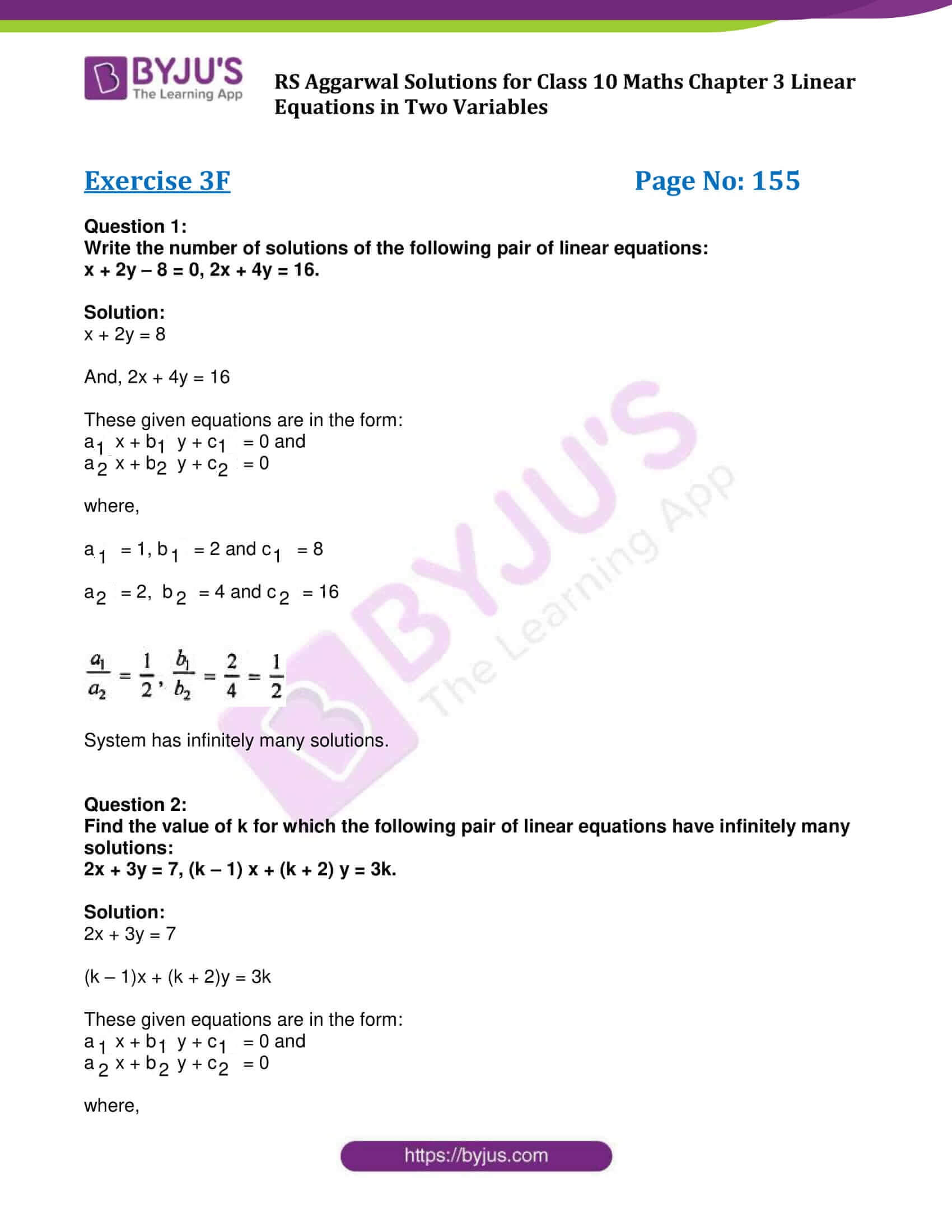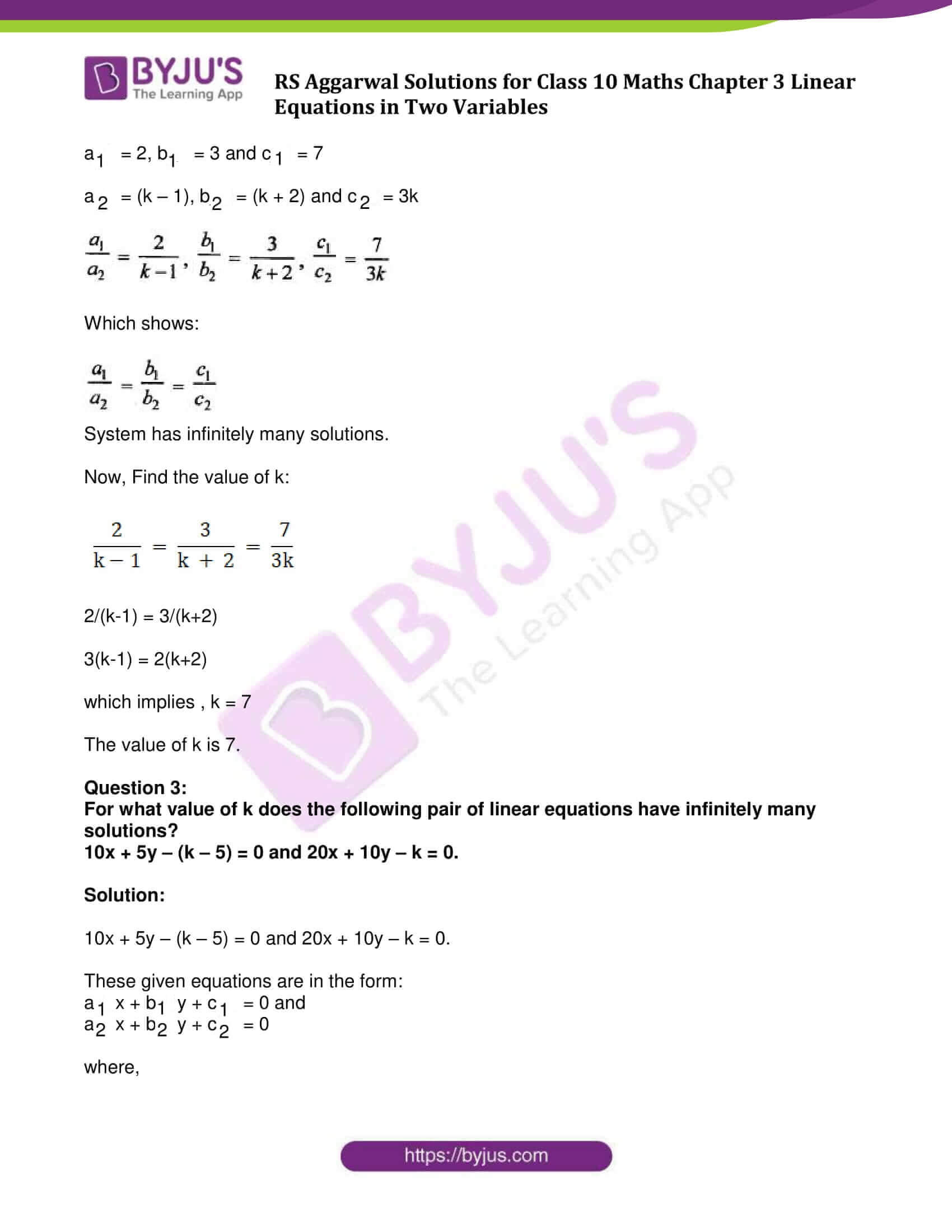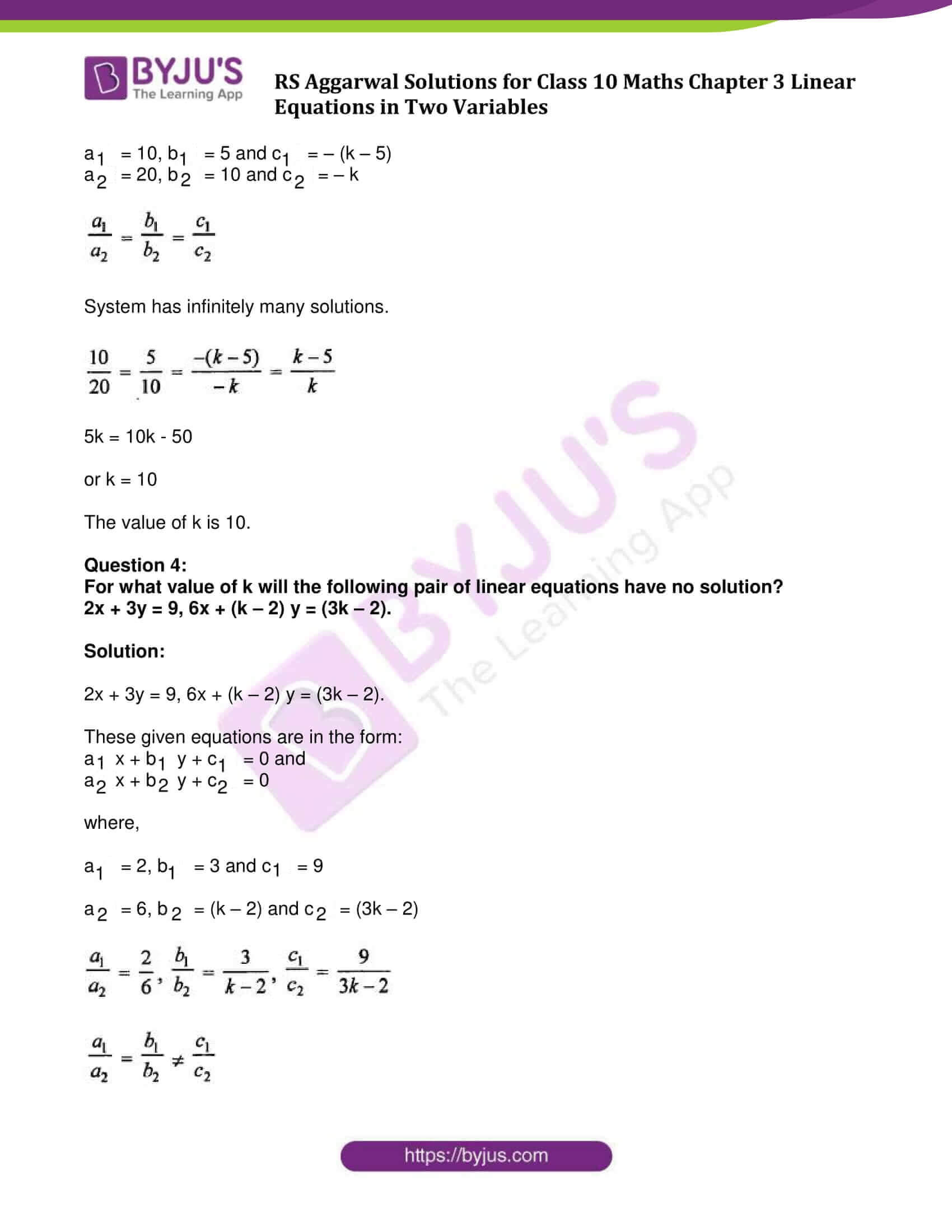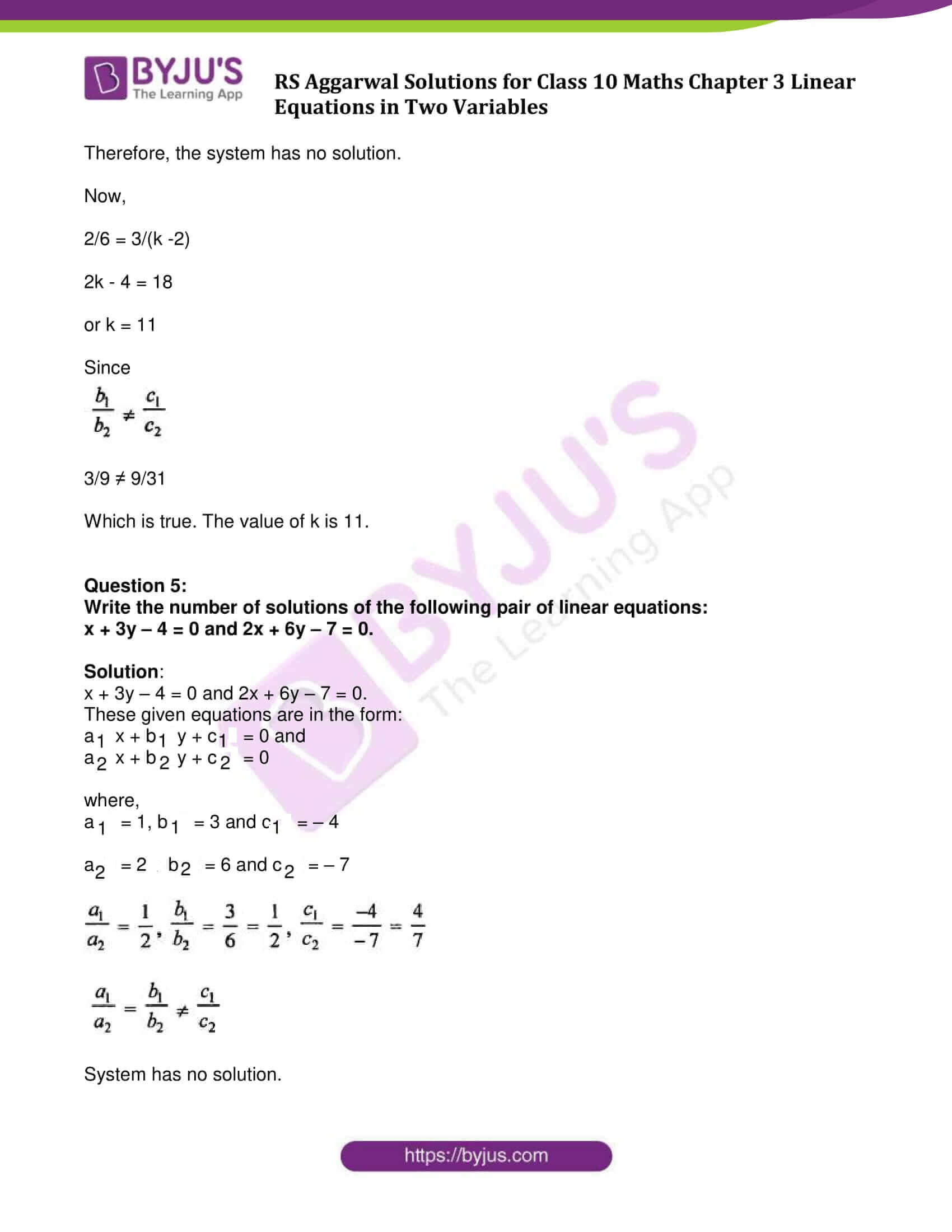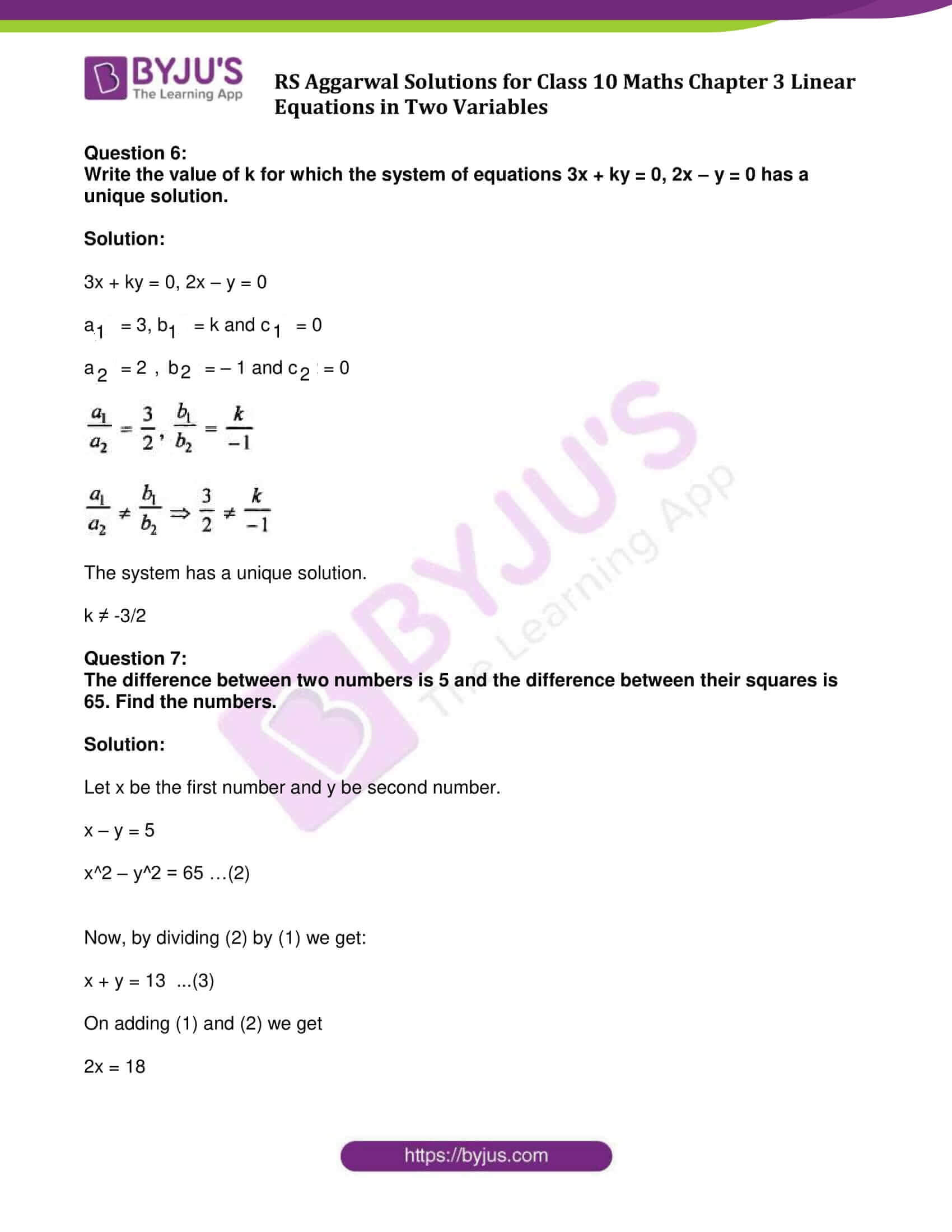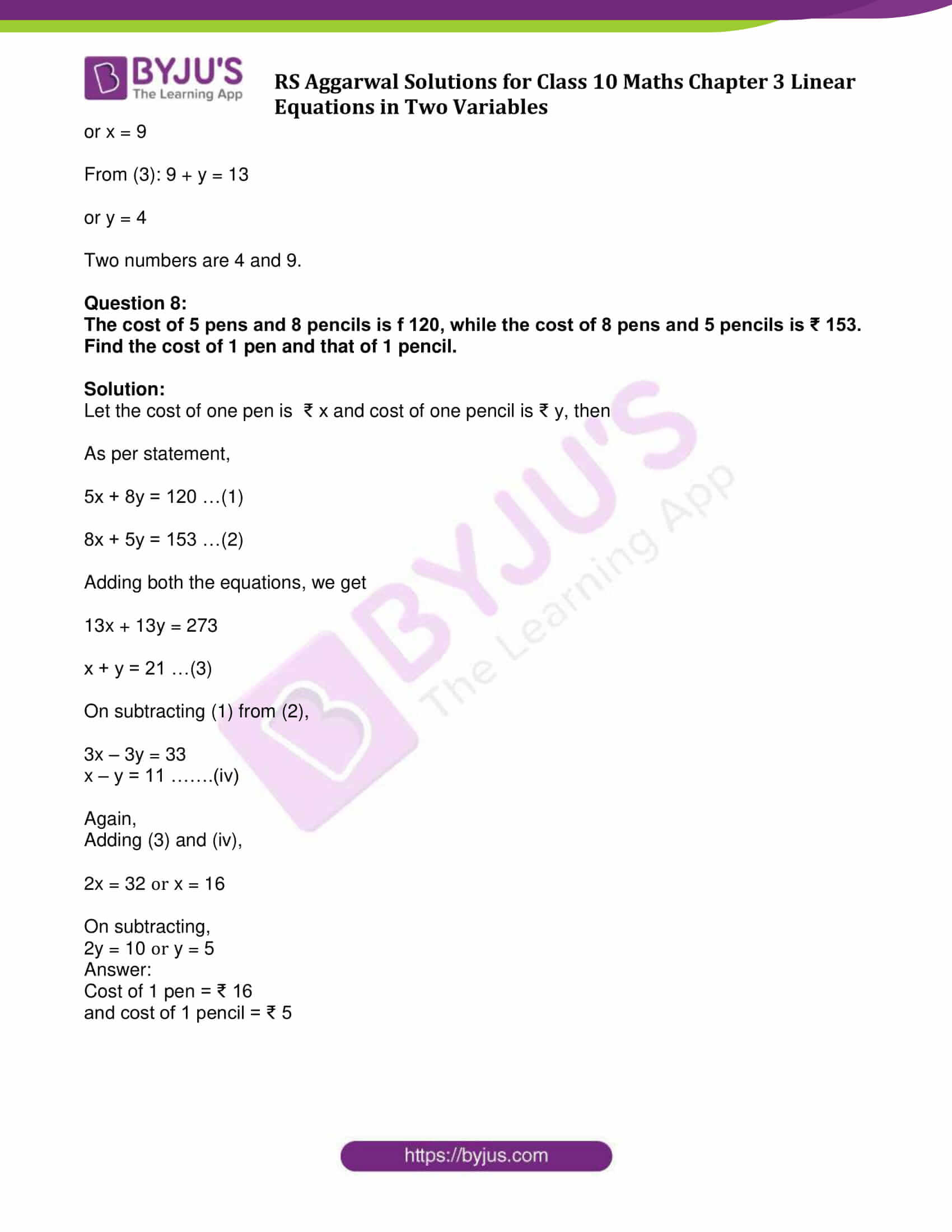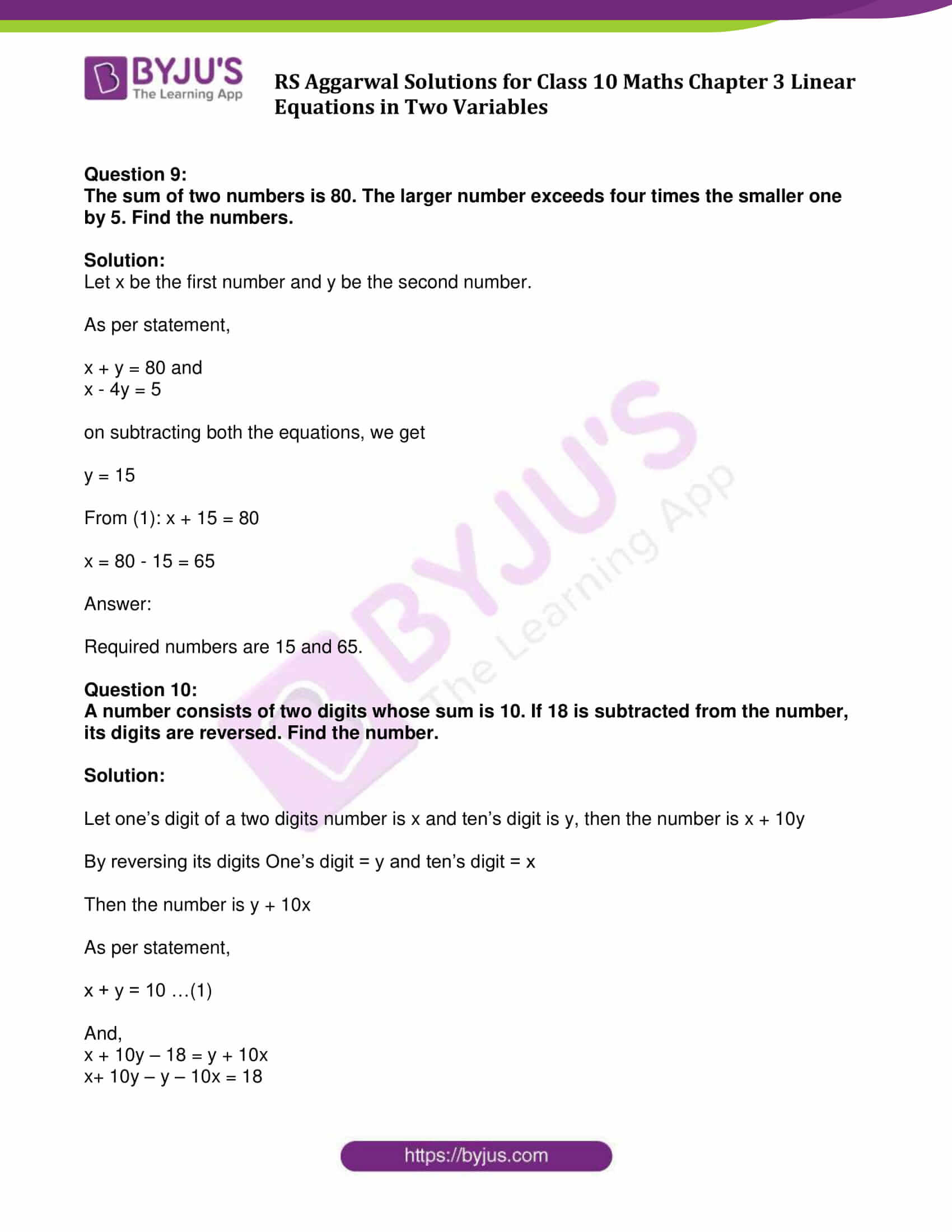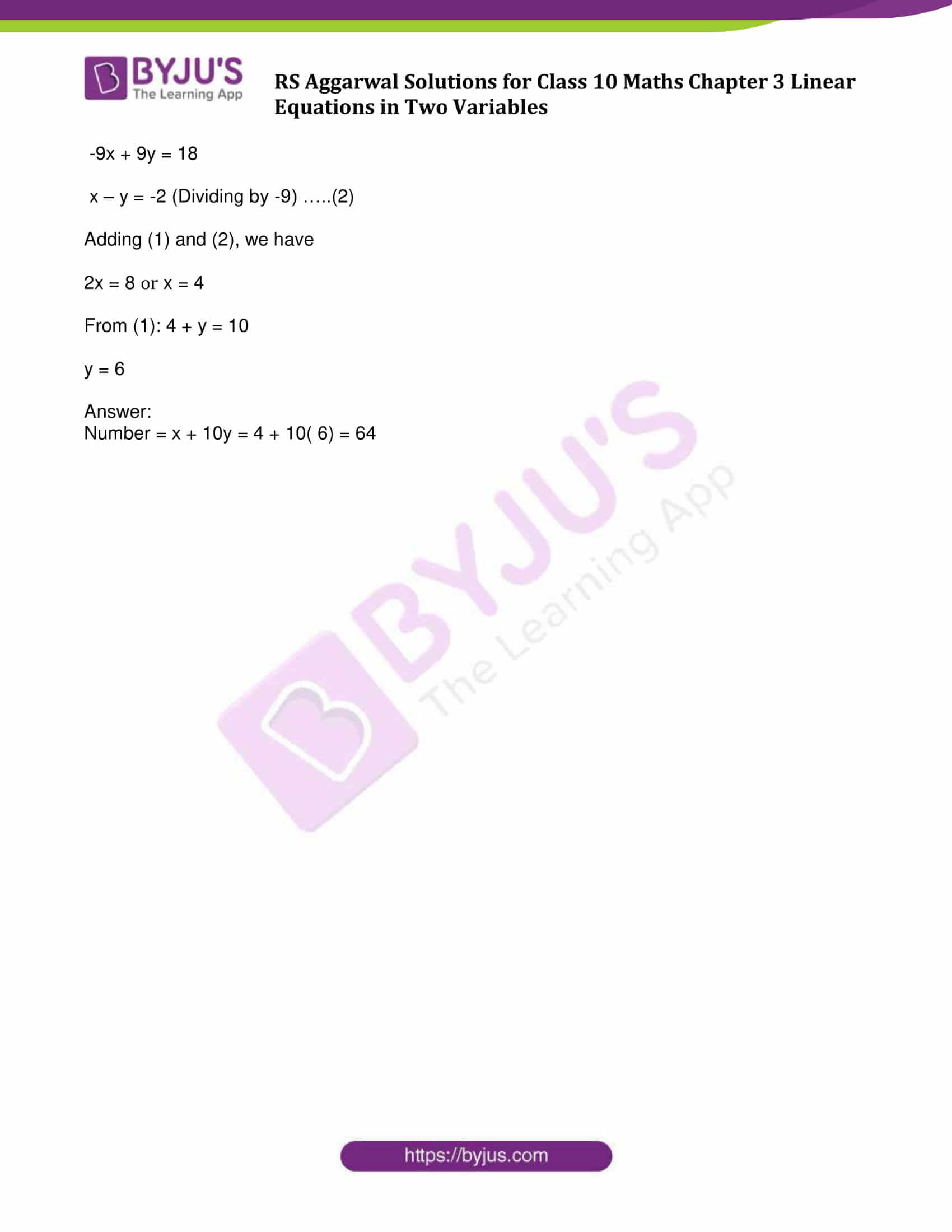### Access other exercise solutions of Class 10 Maths Chapter 3 Linear Equations in Two Variables

Exercise 3A Solutions: 29 Questions (Long Answers)

Exercise 3B Solutions: 20 Questions (Short Answers)

Exercise 3C Solutions: 13 Questions (Long Answers)

Exercise 3D Solutions: 14 Questions (Short Answers)

Exercise 3E Solutions: 20 Questions (Long Answers)

## Exercise 3F Page No: 155

Question 1:

Write the number of solutions of the following pair of linear equations:

x + 2y – 8 = 0, 2x + 4y = 16.

Solution: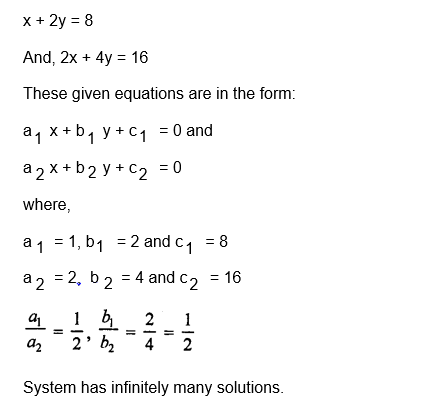Question 2:

Find the value of k for which the following pair of linear equations have infinitely many solutions:

2x + 3y = 7, (k – 1) x + (k + 2) y = 3k.

Solution:

2x + 3y = 7

(k – 1)x + (k + 2)y = 3k

These given equations are in the form:

a1x + b1y + c1 = 0 and

a2x + b2y + c2 = 0

where,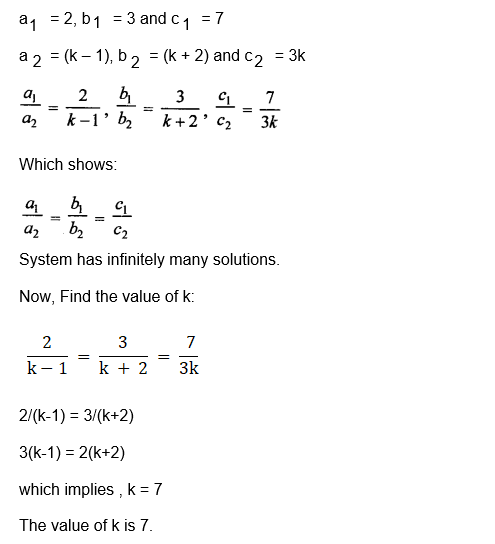Question 3:

For what value of k does the following pair of linear equations have infinitely many solutions?

10x + 5y – (k – 5) = 0 and 20x + 10y – k = 0.

Solution:

10x + 5y – (k – 5) = 0 and 20x + 10y – k = 0.

These given equations are in the form:

a1x + b1y + c1 = 0 and

a2x + b2y + c2 = 0

where,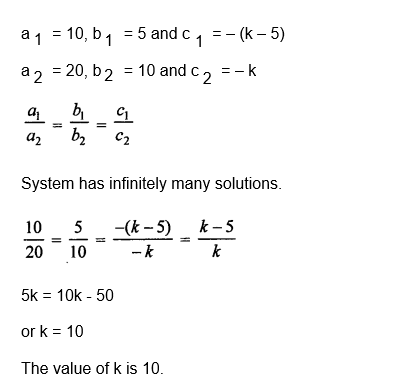Question 4:

For what value of k will the following pair of linear equations have no solution?

2x + 3y = 9, 6x + (k – 2) y = (3k – 2).

Solution:

2x + 3y = 9, 6x + (k – 2) y = (3k – 2).

These given equations are in the form:

a1x + b1y + c1 = 0 and

a2x + b2y + c2 = 0

where,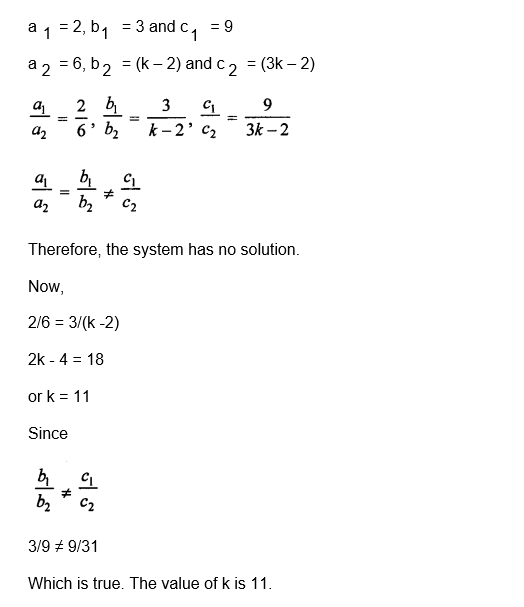Question 5:

Write the number of solutions of the following pair of linear equations:

x + 3y – 4 = 0 and 2x + 6y – 7 = 0.

Solution:

x + 3y – 4 = 0 and 2x + 6y – 7 = 0.

These given equations are in the form:

a1x + b1y + c1 = 0 and

a2x + b2y + c2 = 0

where,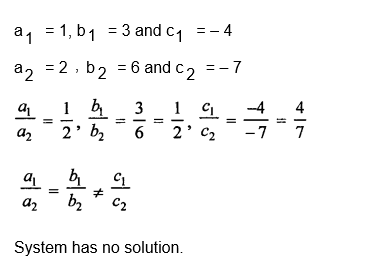Question 6:

Write the value of k for which the system of equations 3x + ky = 0, 2x – y = 0 has a unique solution.

Solution: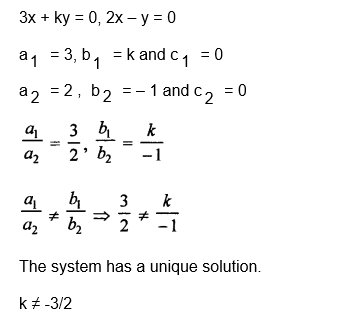Question 7:

The difference between two numbers is 5 and the difference between their squares is 65. Find the numbers.

Solution:

Let x be the first number and y be second number.

x – y = 5

x^2 – y^2 = 65 …(2)

Now, by dividing (2) by (1) we get:

x + y = 13 …(3)

On adding (1) and (2) we get

2x = 18

or x = 9

From (3): 9 + y = 13

or y = 4

Two numbers are 4 and 9.

Question 8:

The cost of 5 pens and 8 pencils is f 120, while the cost of 8 pens and 5 pencils is ₹ 153. Find the cost of 1 pen and that of 1 pencil.

Solution:

Let the cost of one pen is ₹ x and cost of one pencil is ₹ y, then

As per statement,

5x + 8y = 120 …(1)

8x + 5y = 153 …(2)

Adding both the equations, we get

13x + 13y = 273

x + y = 21 …(3)

On subtracting (1) from (2),

3x – 3y = 33

x – y = 11 …….(iv)

Again,

2x = 32 or x = 16

On subtracting,

2y = 10 or y = 5

Cost of 1 pen = ₹ 16

and cost of 1 pencil = ₹ 5

Question 9:

The sum of two numbers is 80. The larger number exceeds four times the smaller one by 5. Find the numbers.

Solution:

Let x be the first number and y be the second number.

As per statement,

x + y = 80 and

x – 4y = 5

on subtracting both the equations, we get

y = 15

From (1): x + 15 = 80

x = 80 – 15 = 65

Required numbers are 15 and 65.

Question 10:

A number consists of two digits whose sum is 10. If 18 is subtracted from the number, its digits are reversed. Find the number.

Solution:

Let one’s digit of a two digits number is x and ten’s digit is y, then the number is x + 10y

By reversing its digits One’s digit = y and ten’s digit = x

Then the number is y + 10x

As per statement,

x + y = 10 …(1)

And,

x + 10y – 18 = y + 10x

x+ 10y – y – 10x = 18

-9x + 9y = 18

x – y = -2 (Dividing by -9) …..(2)

Adding (1) and (2), we have

2x = 8 or x = 4

From (1): 4 + y = 10

y = 6

Number = x + 10y = 4 + 10( 6) = 64

## RS Aggarwal Solutions for Class 10 Maths Chapter 3 Linear Equations in Two Variables Exercise 3F

Class 10 Maths Chapter 3 Linear Equations in Two Variables Exercise 3f is based on the topic and subtopics:

• Solution of a linear equation
• Simultaneous linear equations in two variables
• Solution of a given system of two Simultaneous equations
• Consistent and Inconsistent systems of linear equations
• Solving simultaneous linear equations using graphical method
• Solving simultaneous linear equations using algebraic methods
• Conditions for solvability of linear equations
• Word problems on linear equations